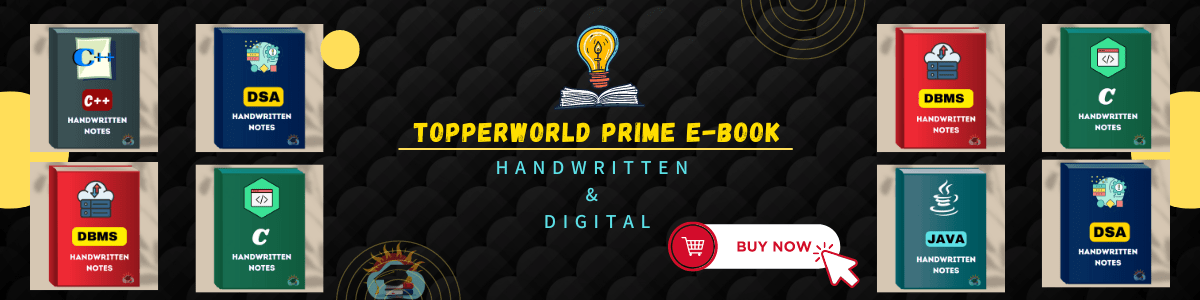### DSA Handwritten Notes

Data structure and algorithm ( DSA ) are two critical concepts in computer science and software development. Data structure is a way of organizing and storing data in a computer so that it can be accessed and used efficiently. On the other hand, an algorithm is a set of instructions or steps used to solve a particular problem or perform a specific task. In this post, we will explore the relationship between data structure and algorithm, and why they are essential for software development.
Data structures and algorithms ( DSA ) are closely related because the choice of data structure affects the efficiency of the algorithm. For example, if we use an array to implement a stack, the push and pop operations will be efficient because they can be done in constant time. However, if we use a linked list to implement a stack, the push and pop operations will be less efficient because they involve traversing the linked list.

This PDF contain Handwritten Notes of Data Structure and Algorithm (DSA).The content inside the DSA PDF are organized in such a way that it is useful for both academic as well as interview purpose.

#### Topics covered In this PDF :

• DS Introduction
• DS Algorithm
• Asymptotic Analysis
• DS Pointer
• DS Structure
• DS Array
• DS Array2D Array
• Circular Doubly List
• Skip list in DS
• DS Stack
• DS Stack
• Array Implementation
• DS Queue
• DS Queue
• Types of Queues
• Array Representation
• Circular Queue
• Deque
• Priority Queue
• Binary Tree
• Binary Search Tree
• etc….## Java Handwritten Notes

Topics Covered In this Book

## C Language Handwritten Notes

This books contain Handwritten Notes

## Python Handwritten Notes

This books contain Handwritten Notes

## C++ Complete Notes

This books contain Digital Notes

## DSA Handwritten Notes

This books contain Handwritten Notes

### 4 thoughts on “DSA Handwritten Notes”

1.2.